# DoubleLi

517712484

:: :: :: :: :: ::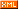:: ::3603 随笔 :: 2 文章 :: 446 评论 :: 0 引用

# 为什么要lambda函数

#include "stdafx.h"
#include <algorithm>   //标准模板库算法库
#include <cmath>       //数学库
#include <iostream>
using namespace std;

//绝对值排序
void abssort(float* x, unsigned n)
{
//模板库排序函数
std::sort(x, x + n,
// Lambda 开始位置
[](float a, float b)
{
return (std::abs(a) < std::abs(b));
} // lambda表达式结束
);
}

int _tmain(int argc, _TCHAR* argv[])
{
float a = { 2.1f, 3.5f, 4.0f, 5.2f, 3.3f };
abssort(a, 5);
for (auto& x : a)
{
cout << x << endl;
}
system("pause");
return 0;
}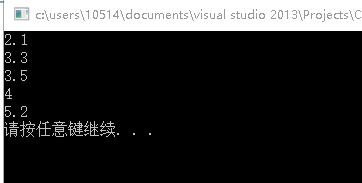# lambda函数的语法

[capture](parameters)->return-type {body}
• []叫做捕获说明符，表示一个lambda表达式的开始。接下来是参数列表，即这个匿名的lambda函数的参数。
• parameters，普通参数列表
• ->return-type表示返回类型，如果没有返回类型，则可以省略这部分。这涉及到c++11的另一特性，参见自动类型推导，最后就是函数体部分。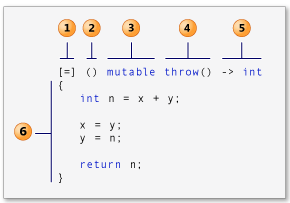• capture clause（捕获）
• lambda-parameter-declaration-list （变量列表）
• mutable-specification （捕获的变量可否修改）
• exception-specification （异常设定）
• lambda-return-type-clause （返回类型）
• compound-statement （函数体）

[&total, factor]
[&, factor]
[=, &total]

#include <functional>
#include <iostream>
using namespace std;int _tmain(int argc, _TCHAR* argv[])
{
//lambd函数对象
auto fl = [](int x, int y){return x + y; };
cout << fl(2, 3) << endl;

function<int(int, int)>f2 = [](int x, int y){return x + y; };
cout << f2(3, 4) << endl;
system("pause");
return 0;
}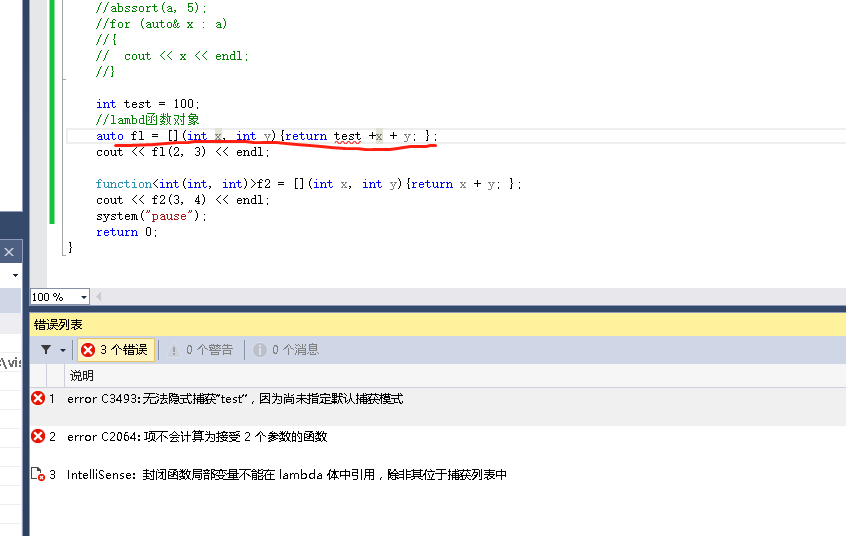int test = 100;
//lambd函数对象捕获局部变量
auto fl = [test](int x, int y){return test +x + y; };
cout << fl(2, 3) << endl;

int test = 100;
//lambd函数对象捕获所有局部变量
auto fl = [=](int x, int y){return test +x + y; };
cout << fl(2, 3) << endl;

## 捕获外部变量

[captures] (params) mutable-> type{...} //lambda 表达式的完整形式

• [ ]        ：无捕获，函数体内不能访问任何外部变量
• [ =]      ：以值（拷贝）的方式捕获所有外部变量，函数体内可以访问，但是不能修改。
• [ &]      ：以引用的方式捕获所有外部变量，函数体内可以访问并修改（需要当心无效的引用）；
• [ var]   ：以值（拷贝）的方式捕获某个外部变量，函数体可以访问但不能修改。
• [ &var] ：以引用的方式获取某个外部变量，函数体可以访问并修改
• [ this]   ：捕获this指针，可以访问类的成员变量和函数，
• [ =，&var] ：引用捕获变量var，其他外部变量使用值捕获。
• [ &，var]：只捕获变量var，其他外部变量使用引用捕获。

int x = 0,y=0;
• auto f1 = [=](){ return x; };                       //以值方式捕获使用变量，不能修改
• auto f2 = [&](){ return ++x; };                  //以引用方式捕获所有变量，可以修改，但要当心引用无效
• auto f3 = [x](){ return x; };                      //以值方式捕获x，不能修改
• auto f4 = [x,&y](){ y += x; };                    //以值方式捕获x，以引用方式捕获y，y可以修改
• auto f5 = [&,y](){ x += y;};                      //以引用方式捕获y之外所有变量，y不能修改
• auto f6 = [&](){ y += ++x;};                    //以引用方式捕获所有变量，可以修改
• auto f7 = [](){ return x ;};                      //无捕获，不能使用外部变量，编译错误

• f1();                     //以值方式捕获，x,y不发生变化
• f2();                    //函数内部x值为0，之后变为1，y没有被修改，值仍然是0；
• f3();                    //函数内部x值仍然为0，即f3()==0;
• f4();                    //x,y均是0，运算后y仍然是0；
• f5();                    //y是0；引用捕获的x是1，运算后x仍然为1；
• f6();                   //x,y均引用捕获，运算后x,y均是2

 1 2 3 4 5 6 7 8 9 10 11 12 13 14 15 16 17 18 19 20 21 #include  #include    int main() {    using namespace std;      int i = 3;    int j = 5;      // The following lambda expression captures i by value and    // j by reference.    function<int (void)> f = [i, &j] { return i + j; };      // Change the values of i and j.    i = 22;    j = 44;      // Call f and print its result.    cout << f() << endl; }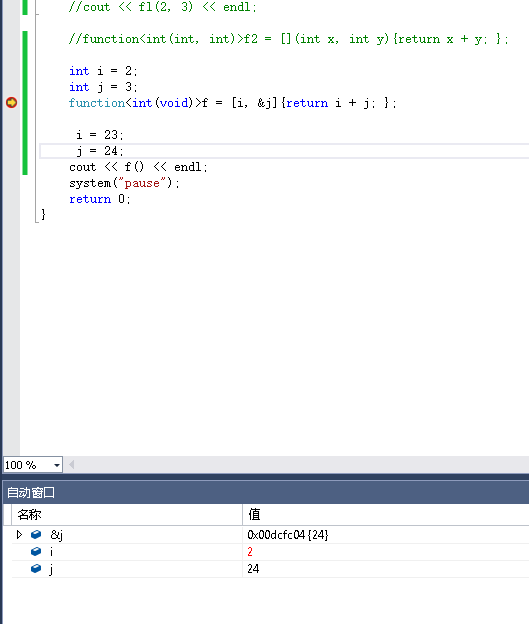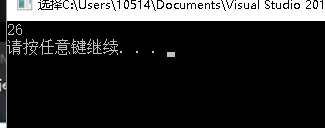• ## 把lambda表达式当作参数传送

#include <list>
#include <algorithm>
#include <iostream>
int main()
{
using namespace std;
// Create a list of integers with a few initial elements.
list<int> numbers;
numbers.push_back(13);
numbers.push_back(17);
numbers.push_back(42);
numbers.push_back(46);
numbers.push_back(99);

// Use the find_if function and a lambda expression to find the
// first even number in the list.
const list<int>::const_iterator result =
find_if(numbers.begin(), numbers.end(),[](int n) { return (n % 2) == 0; });//查找第一个偶数

// Print the result.
if (result != numbers.end())
{
cout << "The first even number in the list is " << *result << "." << endl;
} else
{
cout << "The list contains no even numbers." << endl;
}
}

• ## lambda表达式嵌套使用

#include <iostream>

int main()
{
using namespace std;

// The following lambda expression contains a nested lambda
// expression.
int timestwoplusthree = [](int x) { return [](int y) { return y * 2; }(x) + 3; }(5);

// Print the result.
cout << timestwoplusthree << endl;
}

• ## ambda表达式使用在高阶函数里

#include <iostream>
#include <functional>

int main()
{
using namespace std;

auto addtwointegers = [](int x) -> function<int(int)> {
return [=](int y) { return x + y; };
};

auto higherorder = [](const function<int(int)>& f, int z) {
return f(z) * 2;
};

// Call the lambda expression that is bound to higherorder.
}